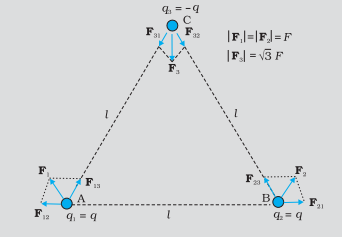Home/Class 12/Physics/

Consider the charges $$q, q$$ and $$-q$$ placed at the vertices of an equilateral triangle, as shown in figure. What is the force on each charge?Speed
00:00
05:25## QuestionPhysicsClass 12

Consider the charges $$q, q$$ and $$-q$$ placed at the vertices of an equilateral triangle, as shown in figure. What is the force on each charge?By observing the figure
Force acting on charge $$q$$ at $$A$$ due to charge $$q$$ at $$B$$ is $$F_{12}$$ along $$\mathit{BA}$$ as both charges are of same nature therefore repulsive in nature.
Force acting on charge $$q$$ at $$A$$ due to charge $$-q$$ at $$C$$ is $$F_{13}$$ along $$\mathit{AC}$$ as both charges are opposite in nature therefore attracts each other.
By the law of parallelogram, the total force $$F_1$$ on the charge $$q$$ at $$A$$ can be found as,
$$F_1=Fȓ_1$$
where as $$ȓ_1$$ is a unit vector along $$\mathit{BC}$$ which is at an angle of $$120^{\circ}$$ from $$x$$- axis .
The force of attraction or repulsion for each pair of charges $$($$either $$\mathit{AB}$$ or $$\mathit{BC}$$ or $$\mathit{CA})$$ has the same magnitude and therefore,
$$F=\frac 1{4\pi \varepsilon _0}\frac{q^2}{l^2}$$
where the magnitude of charge is $$q$$ and the distance between each pair is $$l$$ .
The total force $$F_2$$ on charge $$q$$ at $$B$$ is therefore $$F_2=Fȓ_2$$ , where $$ȓ_2$$ is a unit vector along $$\mathit{AC}$$ .
And the total force on charge $$-q$$ at $$C$$ is $$F_3=\sqrt 3F\widehat n$$ , where $$\widehat n$$ is the unit vector along the direction bisecting the $${\angle}\mathit{BCA}$$ .